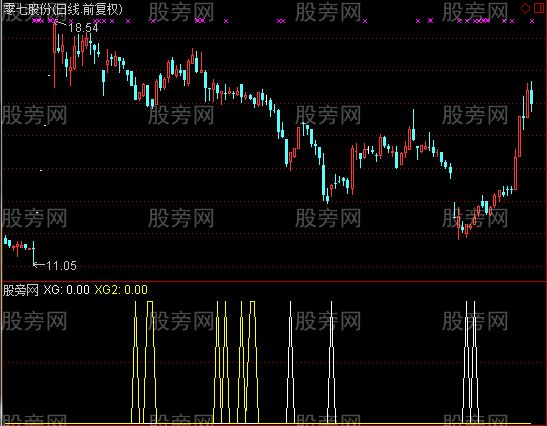# 通达信RSI新用选股指标公式

LC:=REF(CLOSE,1);
RSI1:=SMA(MAX(CLOSE-LC,0),8,1)/SMA(ABS(CLOSE-LC),8,1)*100;
RSI2:=SMA(MAX(CLOSE-LC,0),55,1)/SMA(ABS(CLOSE-LC),55,1)*100;
XG:CROSS(RSI1,20) AND RSI2<=49;
XG2:RSI1>20 AND RSI1<40 AND RSI2>52;